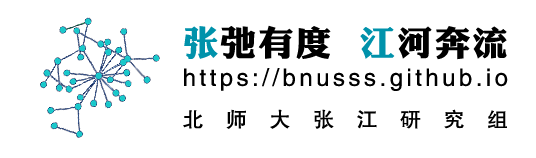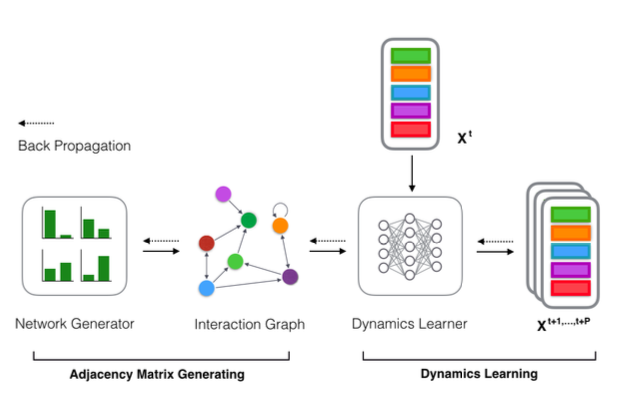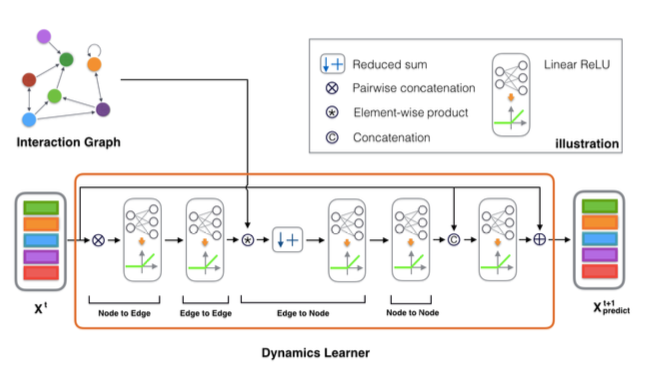# Deep Learning based method for Network Reconstruction

## Paper

The preview PDF has been published in arxiv: A General Deep Learning Framework for Network Reconstruction and Dynamics Learning

Abstract

Introduction

GGN Architecture

## Abstract

Many complex processes can be viewed as dynamical systems on networks. However, in real cases, only the performances of the system are known, the network structure and the dynamical rules are not observed. Therefore, recovering latent network structure and dynamics from observed time series data are important tasks because it may help us to open the black box, and even to build up the model of a complex system automatically. Although this problem hosts a wealth of potential applications in biology, earth science, and epidemics etc., conventional methods have limitations. In this work, we introduce a new framework, Gumbel Graph Network (GGN), which is a model-free, data-driven deep learning framework to accomplish the reconstruction of both network connections and the dynamics on it. Our model consists of two jointly trained parts: a network generator that generating a discrete network with the Gumbel Softmax technique; and a dynamics learner that utilizing the generated network and one-step trajectory value to predict the states in future steps. We exhibit the universality of our framework on different kinds of time-series data: with the same structure, our model can be trained to accurately recover the network structure and predict future states on continuous, discrete, and binary dynamics, and outperforms competing network reconstruction methods.

## Introduction

Many complex processes can be viewed as dynamical systems on an underlying network structure. Network with the dynamics on it is a powerful approach for modeling a wide range of phenomena in real-world systems, where the elements are regarded as nodes and the interactions as edges[1–3]. One particular interest in the field of network science is the interplay between the network topology and its dynamics. Much attention has been paid on how collective dynamics on net- works are determined by the topology of graph. However, in real cases, only the performances, i.e., the time series of nodes states are observed, but the network structure and the dynamical rules are not known. Thus, the inverse problems, i.e., inferring network topology and dynamical rules based on the observed dynamics data, is more significant. This may pave a new way to detect the internal structure of a system according to its behaviors. Furthermore, it can help us to build up the dynamical model of a complex system according to the observed performance automatically.
For example, inferring gene regulatory networks from expression data can help us to identify the major genes and reveal the functional properties of genetic networks; in the study of climate changes, network reconstruction may help us to reveal the atmospheric teleconnection patterns and understand their underlying mechanisms; it can also find applications in reconstructing epidemic spreading processes in social networks, which is essential to identifying the source and pre- venting further spreading. Furthermore, if not only the network structure but also the dynamics can be learned very well for these systems, surrogate models of the original problems can be obtained, on which, many experiments that are hard to implement on the original systems can be operated. Another potential applica- tion is automated machine learning (AutoML)[8, 9]. At present, the main research problem of Neural Architecture Search(NAS), a sub-area of AutoML, is to find the optimal neural network architecture in a space by the search strategy, and it is essentially a network reconstruction problem, in which the optimal neural network and the dynamical rules on it can be learned according to the observed training samples as time series. In a word, reconstructions of network and dynamical rules are pivotal to a wide span of applications.
A considerable amount of methods have been proposed for reconstructing net- work from time series data. One class of them is based on the method of statistical inference such as Granger causality[10, 11], and correlation measurements[12–14]. These methods, however, can usually discover functional connectivity and may fail to reveal structural connection . This means that in the reconstructed system, strongly correlated areas in function need to be also directly connected in struc- ture. Nevertheless this requirement is seldom satisfied in many real-world systems like brain  and climate systems . Another class of methods were developed for reconstructing structural connections directly under certain assumptions. For example, methods such as driving response or compressed sensing[7, 18–20] ei- ther require the functional form of the differential equations, or the target specific dynamics, or the sparsity of time series data. Although a model-free framework presented by Casadiego et al. do not have these limitations, it can only be ap- plied to dynamical systems with continuous variables so that the derivatives can be calculated. Thus, a general framework for reconstructing network topology and learning dynamics from the time series data of various types of dynamics, including continuous, discrete and binary ones, is necessary.
Recently, deep Learning has gained success in many areas such as image classi- fication  and speech recognition . Can we apply this state-of-the-art tech- nique on network reconstruction problem? This is possible because Graph network framework  have enabled deep learning techniques applied on graph structures successfully by mapping graph-structured data onto Euclidean space with update and aggregation functions . With a wealth of different avenues available, GN can be tailored to perform various tasks, such as node or graph classification [26, 27], graph generation [28–31], and spatial-temporal forecasting [32–35]. Recently, the topic of recovering interactions and predicting physical dynamics under given inter- action networks has attracted much attention. A most used approach is introduced by Battaglia et al. , representing particles as nodes and interactions as edges, then reconstruct the trajectories in a inference process on the given graph. How- ever, most of the works in this field have focused on physical reasoning task while few dedicate to solving the inverse problem of network science: revealing network topology from observed dynamics. Some related works [37, 38] attempted to infer implicit interaction of the system to help with the state prediction via observation. But they did not specify the implicit interaction as the network topology of the system, therefore the network reconstruction task remains ignored. Of all literature as we known, only NRI (Neural Relational Inference) model is working on this goal. Nevertheless, only a few continuous dynamics such as spring model and Ku- ramoto model are studied, and discrete processes were never considered. So in the rest of this article, we will take NRI as one of our baselines and will be compared against our own model.

# GGN Architecture

## Framework

The general framework of our model is shown in the figure. The input of the model is the feature of all nodes at time step $t$, and the output of the model is the feature of all nodes in the following $P$ steps. The model consists of two modules, a network generator and a dynamics learner. The job of the generator is to generate an adjacency matrix, and the learner will use the adjacency matrix generated and $X^{t}$(feature of all nodes at time $t$) to predict ${ X }^{ t+1 },…,{ X }^{ t+P }$,(feature of all nodes from time $t+1$ to $t+P$).

The Network Generator module uses the Gumbel softmax trick to generate the adjacency matrix. Details are explained in subsection 3. The goal of the Dynamics Learner is to map the features of all nodes from time $t$ to time $t+1$ through generated adjacency matrix. Similar to NRI’s design\cite{kipf_neural_2018}, our GNN comprises of 4 mapping processes between nodes and edges, which can be accomplished through MLP, CNN or RNN module. In this article, we use MLP. Details are further explained in subsection 4. To learn the complex non-linear process, we use Graph Neural Network instead of Graph Converlutional Network\cite{kipf2016semi}, since the latter does not consider the nonlinear coupling between nodes while sometimes it exists (for example, Kuramoto model).

The temporal complexity and the spatial complexity are both $O(N^2)$.## Network Generator

One of the difficulties for reconstructing a network from the data is the discreteness of the graph, such that the back-propagation technique, which is widely used in differential functions, cannot be applied.
To conquer this problem, we apply Gumbel-softmax trick to reconstruct the adjacency matrix of the network directly. This technique simulates the sampling process from a discrete distribution by a continuous function such that the distributions generated from the sampling processes in real or simulation are identical. In this way, the simulated process allows for back-propagation because it is differentiable.

Network generator is a parameterized module to generate adjacency matrix. Specifically, for a network of $N$ nodes, it uses a $N \times N$ parameterized matrix to determine the $N \times N$ elements in the adjacency matrix $A$, with $\alpha_{ij}$ denoting the probability that $A_{ij}$ takes value 1.
Specifically, the method to generate an adjacency matrix is shown below
where $\xi {ij}$s and $\xi’{ij}$s are i.i.d. random numbers following the gumbel distribution\cite{nadarajah2004beta}. This calculation uses a continuous function with random noise to simulate a discontinuous sampling process. And the temperature parameter $\tau$ adjusts the sharpness of the output. When $\tau\rightarrow 0$, $a{ij}$ will take 1 with probability $\alpha_{ij}$ and 0 with probability $1-\alpha_{ij}$.
Since $\alpha_{ij}$s are all trainable parameters, they can be adjusted according to the back propagation algorithm. Thanks to the features of Gumbel-softmax trick, the gradient information can be back propagated through the whole computation graph although the process of sampling random numbers is non-differentiable.

## Dynamics LearnerLearning with graph-structured data is a hot topic in deep learning research areas. Recently, Graph networks (GNs) \cite{battaglia2018relational} have been widely investigated and have achieved compelling performance in node classification, link prediction, etc. In general, a GN uses the graph structure $A$ and $X^{t}$, which denotes features of all nodes at time $t$, as its input to learn the representation of each node. Specifically, the graph information used here is the adjacency matrix constructed by the generator. The whole dynamics learner can be presented as a function:
\begin{equation} {X}^{t}_{predict}=f({X}^{t-1},A) \end{equation}
where $X^t$ is the state vector of all $N$ nodes at time step $t$, $A$ is the adjacency matrix constructed by the network generator. Similar to the work \cite{kipf_neural_2018}, we realized this function through four mappings operating in succession: Node to Edge, Edge to Edge, Edge to Node and Node to Node, as shown below. Details are explained in the caption of \ref{fig:graphnetwork}.

\begin{figure}[!ht] \centering \includegraphics[width=1\linewidth]{untitled.png} \caption{The Structure of the Dynamics Learner. Dynamics Learner takes the graph structure (here we use Adjacency Matrix) and node states $X$ as its input to predict node states at next time step(s). Four main parts operate in succession to accomplish the whole process: Node to Edge, Edge to Edge, Edge to Node and Node to Node. } \label{fig:graphnetwork} \end{figure}

\begin{equation} H_{ e1 }^{ t-1 }=f_{ v\rightarrow e }( X^{ t-1 }\otimes({ X^{ t-1 }) }^{ T } ) \end{equation}
\begin{equation} H_{ e2 }^{ t-1 }=f_{ e }(H_{ e1 }^{ t-1 }) \end{equation}
\begin{equation} H_{ v1 }^{ t }=f_{ e\rightarrow v }(A * H_{ e2 }^{ t-1 }) \end{equation}
\begin{equation} H_{ v2 }^{ t }=f_{ v }(H_{ v1 }^{ t }) \end{equation}
Where, $H_{.}$ are hidden layers, Operation $\otimes$ is pair-wised concatenation, represented by the formula $\mathbf v \otimes \mathbf v^\top = \left{ \langle \mathbf v_i, \mathbf v_j \rangle \right}{N\times N}$, resulting in a matrix where each element is a node pair. The operation is similar to the Kronecker Product except that we replace the internal multiplication with concatenation. Element-wised product * of Adjacency matrix and the result of Edge to Edge mapping sets elements 0 if there is no connection between two nodes and Reduced sum operation will aggregate edge information to the node.
Finally, we introduce skip-connection in ResNet \cite{He2015} to improve the gradient flow through the network, which enhances the performance of the Dynamics Learner. $X^{ t }$ denotes the nodes’ states at time $t$. $f { output }$ is another MLP. This process can be presented as a function
\begin{equation} X^{ t }{predict}=f{ output }(\left[ X^{ t-1 },H_{ v2 }^{ t } \right] )+X^{ t-1 } \end{equation}
Where $\left[ .,. \right]$ denotes the concatenation operator, note that this operation, as well as the skip-connection trick are optional. We use these method only in experiments on Kuramoto. To make multi-step predictions, we feed in the output states and reiterate until we get the prediction sequence $X_{predict }=({ X }{ predict } ^{ 1 } , … , { X }{predict } ^{ T })$. Then we back propagate the loss between model prediction and the ground truth.